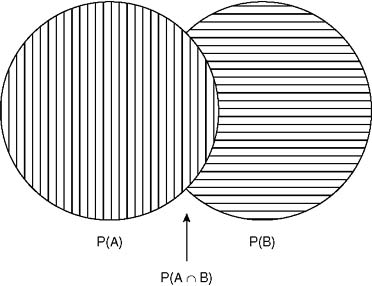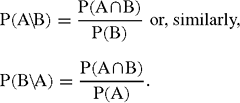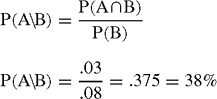Definition: conditional probability from Dictionary of Psychological Testing, Assessment and Treatment

The situation in which the probability that one event will occur is dependent upon another event also occurring (e.g. for there to be a chance of a rainbow, it must first rain).

Summary Article: Conditional Probability
From Encyclopedia of Measurement and Statistics

Conditional probability is a mathematical description of the likelihood that a particular event will take place given the occurrence of a particular precursor event. Thus the probability of occurrence of event A is conditional on the probability of the prior occurrence of event B. Conditional probability is expressed as P (A\B), which is read as “the probability of A given B.” In order to calculate the conditional probability of an event, there must be a priori knowledge of both the probability that the first of the events will occur and the probability that both events will occur together. The basic probabilities of the events involved can be expressed by a Venn diagram, as shown in Figure 1.

In Figure 1, the expressions used are defined as follows:

P (A) is the probability of event A occurring alone

P (B) is the probability of event B occurring aloneFigure 1 Venn Diagram Illustrating the Probabilities of Event A, Event B, and Events A and B Together

P (A∩B) is the probability of both events A and B occurring together, or the joint probability (∩ is the mathematical symbol used to represent the intersection of two sets)

The conditional probability of an event can be calculated by dividing the probability of the occurrence of the first event into the joint probability. The mathematical formula for this calculation isIt follows that the probability of occurrence of the primary event (the denominator of the equation) cannot be equal to zero. Without this condition, the equation would be mathematically impossible, as well as illogical (one cannot estimate the probability of a particular outcome without the occurrence of the outcome's predecessor).

Conditional probability can be applied to many situations in a variety of fields. For example, it could be used to determine a person's chance of developing a particular disease given the disease's presence or absence in the person's familial background or the probability of a person using the word cabbage in a sentence, given that the word directly preceding it was eggplant. For gamblers, conditional probability could be used to compare the odds of winning a game; the chances of having a winning poker hand in five-card draw are significantly greater if a player is dealt three queens than if the player gets a pair of twos. In everyday life, your chances of being mugged in a city might be dependent on how often you walk alone at night, or your chances of heartburn might be conditional on your eating spicy foods.

As an example, suppose there is a 3% chance that a girl in a senior high school class is both a cheerleader and class president. Additionally, suppose that the chance of a girl in the senior class being a cheerleader is 8%. What are the chances of a girl being elected senior class president given that she is a cheerleader?There is a 38% chance that a girl will be elected president of her senior class given that she is a cheerleader.

Conditional probability: http://en.wikipedia.org/wiki/Conditional_probability

• , & (1995). Statistical methods in education and psychology. Boston: Allyn & Bacon.
• (1994). Statistics. Belmont, CA: Wadsworth.

•Copyright © 2007 by SAGE Publications, Inc.

### Related Articles

##### Full text Article Conditional Probability
Encyclopedia of Medical Decision Making

The probability that event E occurs, given that event F has occurred, is called the conditional probability of event E given event F . In...

##### Full text Article Conditional probability
Philosophy of Science A-Z

The probability that (event or outcome) q will happen, on the condition (or given) that p has happened. The (unconditional) probability...

##### Full text Article TOTAL PROBABILITY THEOREM
Encyclopedia of Statistical Sciences

The events E1, E2, …, En are called a partition of the sample space Ω if Ei∩Ej=Ø for all i≠j and . If A⊂Ω is any event, then E1, E2, …, En a

See more from Credo